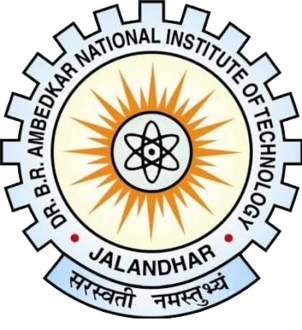# Class Test - Elements Of Mechanical Engineering (Meci 101)

20 Questions | Attempts: 140
ShareSettings1. All question carry equal marks 2. Don't waste time for single question.

• 1.
For a process from state 1 to state 2, heat transfer in a reversible process is given by
• A.

Q for reversible=(To)*(S1-S2)

• B.

Q for reversible=(To)*(S2-S1)

• C.

Q for reversible=(To)/(S1-S2)

• D.

Q for reversible=(To)/(S2-S1)

• 2.
Which of the following is true?
• A.

Q for reversible > Q for irreversible and work for reversible < work for irreversible

• B.

Q for reversible < Q for irreversible and work for reversible > work for irreversible

• C.

Q for reversible < Q for irreversible and work for reversible < work for irreversible

• D.

Q for reversible > Q for irreversible and work for reversible > work for irreversible

• 3.
Which of the following is true for a steady flow system?
• A.

Mass entering = mass leaving

• B.

Mass does not enter or leave the system

• C.

Mass entering can be more or less than the mass leaving

• D.

None of the mentioned

• 4.
The work done by a closed system in a reversible process is always ___ that done in an irreversible process.
• A.

Less than or more than

• B.

Equal to

• C.

Less than

• D.

More than

• 5.
A piston-cylinder contains air at 600 kPa, 290 K and a volume of 0.01m^3. A constant pressure process gives 54 kJ of work out. Find the final volume of the air.
• A.

0.05 m^3

• B.

0.01 m^3

• C.

0.10 m^3

• D.

0.15 m^3

• 6.
A piston-cylinder contains 0.5 kg of air at 500 kPa and 500 K. The air expands in a process so the pressure is linearly decreasing with volume to a final state of 100 kPa and 300 K. Find the work in the process.
• A.

56.1 kJ

• B.

66.1 kJ

• C.

76.1 kJ

• D.

86.1 kJ

• 7.
When a body A is in thermal equilibrium with a body B, and also separately with a body C, then B and C will be in thermal equilibrium with each other.
• A.

True

• B.

False

• 8.
What is the standard fixed point of thermometry?
• A.

The ice point

• B.

The steam point

• C.

The triple point of water

• D.

None of the mentioned

• 9.
Which of the following is chosen as the standard thermometric substance?
• A.

Gas

• B.

Liquid

• C.

Solid

• D.

All of the mentioned

• 10.
Work done by a system is taken to be
• A.

Positive

• B.

Negative

• C.

Zero

• D.

Varies according to situation

• 11.
Work is a
• A.

Point function

• B.

Path function

• C.

Depends on the state

• D.

None of the mentioned

• 12.
The differentials of point functions are
• A.

Perfect differentials

• B.

Exact differentials

• C.

All of the mentioned

• D.

none of the mentioned

• 13.
Constant pressure process is also known as
• A.

Isopiestic process

• B.

Isobaric process

• C.

All of the mentioned

• D.

None of the mentioned

• 14.
Work done in a quasi-static process
• A.

Depends on the path followed

• B.

Independent of the path followed

• C.

Depends only on the initial and final states

• D.

None of the mentioned

• 15.
Shaft uses which kind of motion to do work?
• A.

Vertical motion

• B.

Horizontal motion

• C.

Rotational motion

• D.

None of the mentioned

• 16.
Thermodynamic properties are
• A.

Point function

• B.

Path function

• C.

Depends on the state

• D.

None of the mentioned

• 17.
Cyclic integral of a property is always
• A.

Zero

• B.

One

• C.

Infinite value

• D.

None of the mentioned

• 18.
A real gas behaves as an ideal gas when?
• A.

Temperature approaches zero

• B.

Pressure approaches zero

• C.

Both temperature and pressure approaches zero

• D.

None of the mentioned

• 19.
Heat is a
• A.

Point function

• B.

Path function

• C.

Depends on the state

• D.

None of the mentioned

• 20.
Equation of continuity comes from
• A.

Conservation of energy

• B.

Conservation of mass

• C.

Conservation of work

• D.

Conservation of heat

## Related TopicsBack to top
×

Wait!
Here's an interesting quiz for you.